# Program to check Bouncy Number in Java

In this tutorial you will learn about the Program to check Bouncy Number in Java and its application with practical example.

In this tutorial, we will learn to create a Java Program to check Bouncy Number in Java  using Java programming.

## Prerequisites

Before starting with this tutorial we assume that you are best aware of the following Java programming topics:

• Java Operators.
• Basic Input and Output function in Java.
• Class and Object in Java.
• Basic Java programming. Basic Java programming.
• If-else statements in Java.
• For loop in Java.

Let’s understand first what is increasing and decreasing numbers before what is a Bouncy Number.

## Increasing Number

Increasing number are number their value increases from left-to-right and no digit in right is smaller to its left it is called an increasing number.

example 234.

## Decreasing Number

Decreasing number are number their value decrees from left-to-right and no digit in right is greater to its left it is called an decreasing number.

example 654.

## Bouncy Number

A positive integer that is nor in increasing and nor in decreasing order is called a “bouncy number”

example. 165349

## Program to check Bouncy Number in Java

In this program we will learn to create a program to check Given number is Bouncy Number or not. We would first declared and initialized the required variables. Next, we would prompt user to input a number and later we will find it is bouncy or not.Lets gave a look at the program.

## Output

Bouncy Number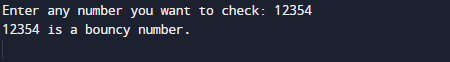Not a Bouncy Number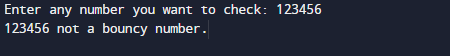In the above program, we have first declared and initialized a set variables required in the program.

Declaring a  method Increasing(). It will checks the given number present in Increasing order or not.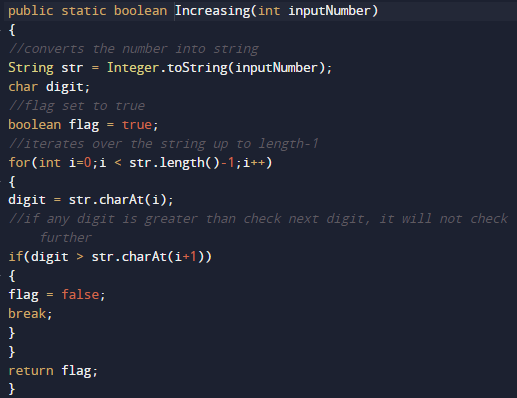Similarly another method  named Decreasing()  it will gives a number in present in Decreasing order or not.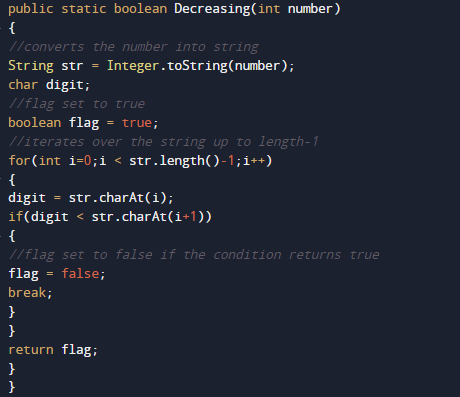After that we check if number not in Increasing order or nor in decreasing order or less than < 101 if any condition became true then the number is nit Bouncy number else number is Bouncy number.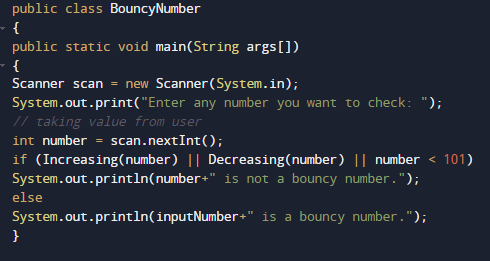So we can say that given number is Bouncy or not.

In this tutorial we have learn about the Program to check Bouncy Number in Java and its application with practical example. I hope you will like this tutorial.